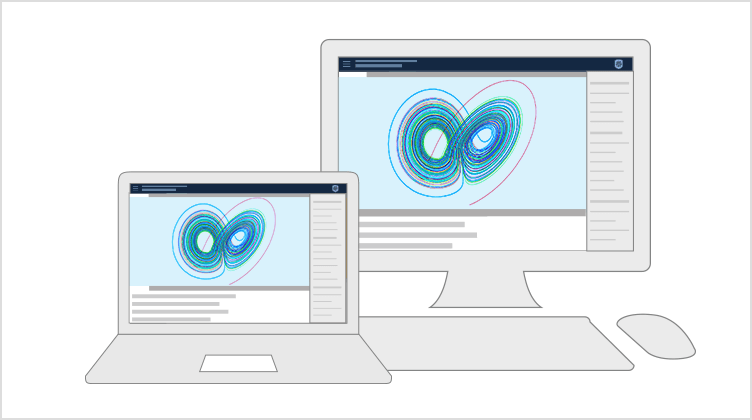#Wolfram U

Interactive Course

# Introduction to Differential Equations

10hr
Intermediate
2 Certifications
Estimated Time: 10hr
Course Level: Intermediate
Prerequisites: This course requires no prior knowledge of Mathematica or the Wolfram Language. Prerequisites for differential equations include calculus and linear algebra.
Certification Levels: Course completion, Wolfram Certified Level I### Summary

A comprehensive introduction to fundamental concepts and solution methods for differential equations, including video lessons and interactive notebooks. Follow along with the examples in the Wolfram Cloud and use the material to prepare for courses in natural science, engineering, economics and other fields. The course starts with a discussion of direction fields and methods for solving first-order differential equations, followed by the study of second-order equations and their applications, and then moves on to solving systems of differential equations. Problem sessions, exercises and quizzes are provided for self-paced assessment. Earn a certificate by watching all lesson and problem session videos and completing the quizzes with a passing grade. Level I certification in Differential Equations is awarded to those who meet the completion requirements and also pass the course final exam.

### You'll Learn To

Visualize direction fields in the plane

Compute series solutions of differential equations

Solve linear systems with constant coefficients

Find exact solutions of differential equations

Use Laplace transforms for solving initial value problems

Apply differential equations to real-world problemsAsk questions or start a discussion about related topics on Wolfram Community.